### Keyword PLOT

This keyword controls the calculation and plotting of molecular fields. See the keywords BOX (4.10.7) and POINTS (4.10.8) for definitions of the plot support.
Options: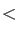FIELD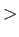Molecular field specification. The available field acronyms are given in Table 12. This specification is mandatory, even though the syntax is that of an option!
BASIS / AUXIS
 BASIS The Kohn-Sham density is used for the construction of the plot function. This is the default. AUXIS The auxiliary function density is used for the construction of the plot function. This option is incompatible with the READ option.
BINARY / ASCII / TABLE
 BINARY A binary file FIELD.bin is written in VU file format. It contains the coordinates and plot function values. The VU control file deMon.pie is written too. This is the default. ASCII An ASCII file FIELD.asc is written. It contains the coordinates and plot function values. TABLE A table with coordinates and plot function values is written to the output file deMon.out. READ Specifies that an orbital list is read in the keyword body of PLOT. This keyword is incompatible with the EFIDS field. LMO Localize molecular orbitals by Cholesky decomposition of the density matrix  before molecular fields are calculated. Only meaningful with the BASIS option. FBLMO Localize molecular orbitals by Foster-Boys method  before molecular fields are calculated. Only meaningful with the BASIS option. PMLMO Localize molecular orbitals by Pipek-Mezey method  before molecular fields are calculated. Only meaningful with the BASIS option. DD The deformation density is used for the molecular field calculation.
Description:
Definition of the molecular field is mandatory for the PLOT keyword, even though the syntax is that of an option. The available molecular fields are listed in Table 12. If the BOX and POINTS keywords are omitted, the default BOX setting is used for the plot. Only one molecular field at a time can be calculated. If several fields are to be calculated, restart calculations with SCFTYPE MAX=0 and GUESS RESTART (see 4.5.1 and 4.5.5) may be done. In any case, no more than 20 molecular field entries at a time can be calculated. A field along with its first and second derivatives counts as 10 entries (1 + 3 + 6).

Table 12: Molecular fields available in deMon2k

 Acronym Description PSI Molecular orbitals. By default, the five highest energy occupied and five lowest energy unoccupied orbitals are calculated. D1PSI Molecular orbitals and first derivatives. By default, the HOMO and LUMO are calculated. D2PSI Molecular orbitals, first, and second derivatives. By default the HOMO is calculated. FUKUI Fukui function matrices. Only valid with the keyword FUKUI. RHO Electron density. By default, the density for the occupied orbitals is calculated. D1RHO Electron density and first spatial derivatives. By default, the density and its derivatives for the occupied molecular orbitals are calculated. D2RHO Electron density, first, and second spatial derivatives. By default, the density and its derivatives for the occupied orbitals are calculated. PRHO Perturbed densities in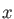,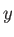, and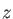electric field directions. SPIN Spin density. By default, the spin density for the occupied molecular orbitals is calculated. D1SPIN Spin density and first spatial derivatives. By default, the spin density and its derivatives for the occupied molecular orbitals are calculated. D2SPIN Spin density, first, and second spatial derivatives. By default, the spin density and its derivatives for the occupied orbitals are calculated. LAP Electron density Laplacian. By default, the Laplacian of the occupied molecular orbitals is calculated. ESP Molecular electrostatic potential. By default, the MESP of the occupied molecular orbitals is calculated. A minus sign in front indicates that only the electronic part is calculated. D1ESP Molecular electrostatic potential and its first derivatives. By default, the MEP and its derivatives of the occupied orbitals are calculated. D2ESP Molecular electrostatic potential and its first and second derivatives. By default, the MEP and its derivatives of the occupied orbitals are calculated. EFI Molecular electric field. By default, the electric field of the occupied molecular orbitals is calculated. D1EFI Molecular electric field and its first derivatives. By default, the electric field and its derivatives of the occupied orbitals are calculated. EFG Molecular electric field gradients. Identical to D1EFI. EFIDS Anion surface generation . ELF Electron localization function [278,279]. ITELF Information theory derived electron localization function . IMF Induced magnetic field and NICS. SGGA Dimensionless density gradientas defined in the PBE functional.

The BASIS and AUXIS options specify the density (Kohn-Sham or auxiliary function density, respectively) used for the calculation of the molecular field. The AUXIS option is incompatible with the orbital specification by the READ option. Use of the auxiliary function density for the calculation of the molecular field will yield a considerable speedup. However, the field values may show deviations of as much as 10% compared to the Kohn-Sham density results. Most certainly these deviations depend on the chosen auxiliary function set (see 4.3.3). In general, the field topology obtained with the auxiliary function density is qualitatively correct.

The PLOT keyword produces two additional output files, the binary file FIELD.bin and the VU control file deMon.pie. With these files, a VU  session can be started. With the ASCII option, only the file FIELD.asc is created. The coordinates and molecular field values are listed in this file. With the TABLE option, the output is created but written to the deMon.out output file.

A set of molecular orbitals can be specified with the READ option for the calculation of molecular fields. However, this option cannot be used for the EFIDS field. In the case of the PSI and D1PSI fields, the READ option specifies the calculated molecular orbitals directly. Again, for PSI no more than 20 orbitals at a time can be calculated and, for D1PSI, no more than 5. If orbitals are selected in the case of other fields, then those fields are constructed from the sum of the selected orbitals. For example the following input specifies the calculation of the density using the molecular orbitals 1, 2, and 4:


1 2 4


Virtual orbitals can be included. They will be occupied by one electron. In the case of an unrestricted calculation (UKS option of keyword SCFTYPE; see 4.5.1),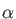and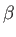-orbitals can be selected, each set in a separate input line. In the following example, the-orbitals 1, 2, 3, 4, 5, 6, 7, and the-orbitals 1, 2, 3, 4, 5, 6 are selected for the calculation of the molecular electrostatic potential:


1 2 3 4 5 6 7
1 2 3 4 5 6


If only the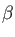-orbitals are to be selected, the first line in the preceding example must have a 0 entry, as follows:


0
1 2 3 4 5 6


With the options LMO, FBLMO, and PMLMO the localization of molecular orbitals is requested. These localized orbitals can either be plotted (in combination with the PSI field option) or used for the calculation of molecular fields. Again, fields can be calculated with subsets of localized orbitals. For the electrostatic potential and its derivatives, it is also possible to plot only the electronic part by using minus signs in front of the corresponding field acronyms, i.e. -ESP, -D1ESP, and -D2ESP. Figure 14 depicts some molecular field plots obtained with the PLOT keyword and rendered with VU .

Figure 14: Plots of spin density for Cr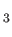, (top left), dimensionless exchange gradient for two C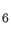H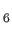(top right) and for B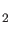H, (bottom left), and IT-ELF for C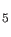H(bottom right).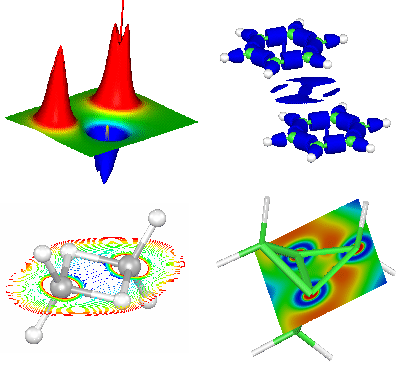The DD option of the PLOT keyword triggers the use of deformation densities calculated according to Eq. (4.28). The deformation density itself is plotted by:

 PLOT RHO DD


With the IMF PLOT option the NICS, the induced magnetic field vectors, and the absolute induced magnetic field values at each point of the grid are calculated. The external magnetic field can be specified with the READ option:

 PLOT IMF READ
1.0 0.0 0.0
#
Geometry
C1    0.000000    1.396400    0.000000
C2    0.000000    0.698200    1.209318
C3    0.000000   -0.698200    1.209318
C4    0.000000   -1.396400    0.000000
C5    0.000000   -0.698200   -1.209318
C6    0.000000    0.698200   -1.209318
H1    0.000000    2.479500    0.000000
H2    0.000000    1.239750    2.147310
H3    0.000000   -1.239750    2.147310
H4    0.000000   -2.479500    0.000000
H5    0.000000   -1.239750   -2.147310
H6    0.000000    1.239750   -2.147310


In this example the external field is oriented along the-axis which is perpendicular to the benzene molecule defined in the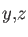-plane. By default the external magnetic field is oriented along the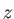-axis.